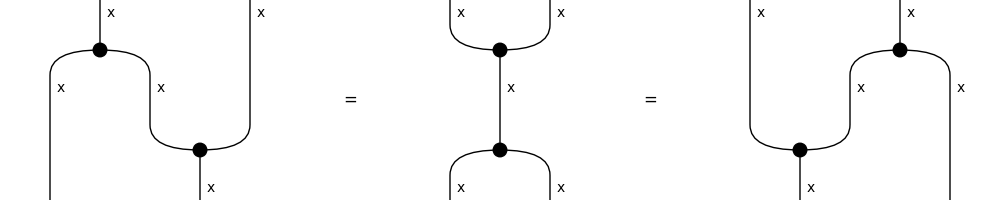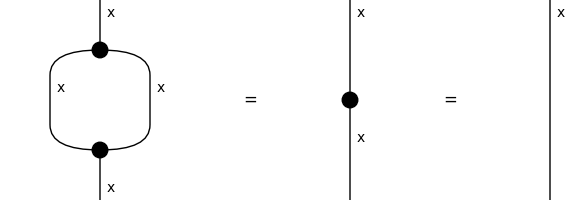# frobenius#

The free symmetric category with a supply of spiders, also known as special commutative Frobenius algebras.

Diagrams in the free hypergraph category are faithfully encoded as `Hypergraph`, see Bonchi et al. [BGK+22].

## Summary#

 `Ob` A frobenius object is a self-dual pivotal object. `Ty` A frobenius type is a pivotal type with frobenius objects inside. `Diagram` A frobenius diagram is a compact diagram and a Markov diagram. `Box` A frobenius box is a compact and Markov box in a frobenius diagram. `Cup` A frobenius cup is a compact cup in a frobenius diagram. `Cap` A frobenius cap is a compact cap in a frobenius diagram. `Swap` A frobenius swap is a compact and Markov swap in a frobenius diagram. `Spider` The spider with `n_legs_in` and `n_legs_out` on a given atomic type, with some optional phase as `data`. `Category` A hypergraph category is a compact category with a method `spiders`. `Functor` A hypergraph functor is a compact functor that preserves spiders.

## Axioms#

```>>> from discopy.drawing import Equation
>>> Diagram.structure_preserving = True
>>> x, y, z = map(Ty, "xyz")
```
```>>> split, merge = Spider(1, 2, x), Spider(2, 1, x)
>>> unit, counit = Spider(0, 1, x), Spider(1, 0, x)
```
• Frobenius:

```>>> frobenius = Equation(
...     split @ x >> x @ merge, merge >> split, x @ split >> merge @ x)
>>> assert frobenius
>>> frobenius.draw(path="docs/_static/frobenius/frobenius.png")
```• Speciality:

```>>> special = Equation(split >> merge, Spider(1, 1, x), Id(x))
>>> assert special
>>> special.draw(path="docs/_static/frobenius/special.png")
```
```>>> Diagram.structure_preserving = False
```